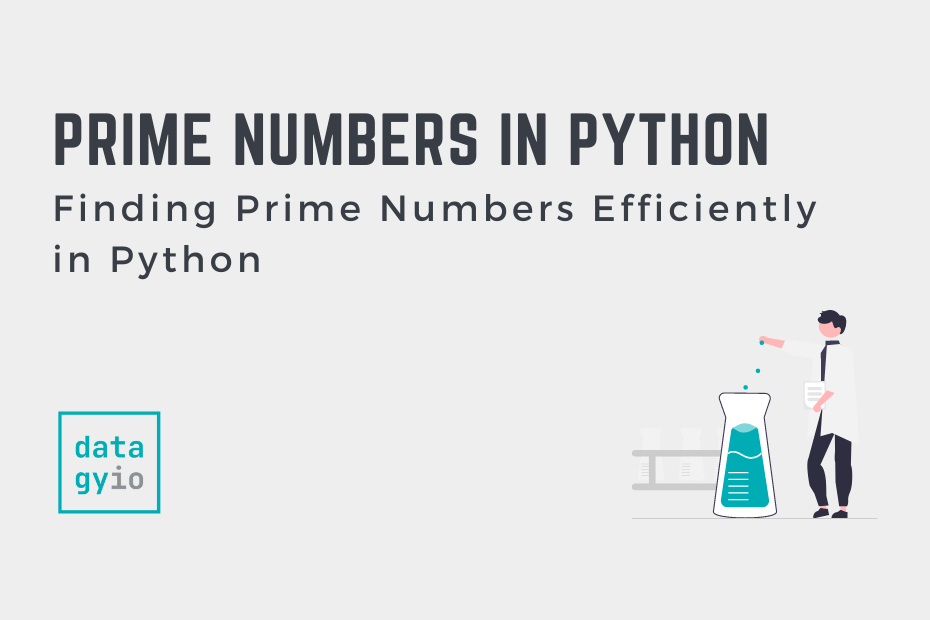# Python Prime Numbers: Find a Value or a Range of ValuesIn this tutorial, you’ll learn how to use Python to find prime numbers, either by checking if a single value is a prime number or finding all prime numbers in a range of values. Prime numbers are numbers that have no factors other than 1 and the number itself. Being able to work with prime numbers is a common task in occupations such as computer and cyber security.

By the end of this tutorial, you’ll have learned:

• What prime numbers are
• Different ways of finding prime numbers in Python
• Optimizing our algorithms for finding prime numbers in Python
• Finding all prime numbers between a range of values in Python

## What are Prime Numbers

Prime numbers are a positive integer that’s greater than 1 that also have no other factors except for 1 and the number itself. For example, the number 5 is a prime number, while the number 6 isn’t (since 2 x 3 is equal to 6).

The first few prime numbers are: 3, 7, 11, 13, etc.

## Finding Prime Numbers in Python (Optimized Code)

Let’s take a look at how we can use Python to determine if a number is a prime number. The most naive and straightforward implementation is to loop over the range of numbers from 2 to the number and see if the modulo of the number and the range is equal to 0. If that occurs, then the number has a divisor other than 1 and the number itself and the number isn’t a prime number.

Let’s take a look at how we can implement this first code:

``````# Writing a Simple Function to Check if a Number is a Prime Number
def is_prime(number):
if number > 1:
for num in range(2, number):
if number % num == 0:
return False
return True
return False

print(is_prime(4))

# Returns: False``````

Let’s break down what we did here:

1. We defined a function `is_prime` that takes a single argument, a number
2. If the number is not greater than 1, then the function returns `False` as prime numbers need to be larger than 1
3. It then loops over the range from 2 through to the number (but not including)
4. If the modulo of the number and the iteration is equal to zero (meaning the number is divided cleanly), then the function returns `False`
5. Otherwise, the function returns `True`

This function works well! However, it’s not the most efficient function. We can divide the number by 2 since any composite number (a non-prime number) will also have a factor of its half.

This means that the number of iterations that our code has to complete is reduced by roughly half! Let’s see how we can write this function:

``````# Improving our function
def is_prime(number):
if number > 1:
for num in range(2, number // 2 + 1):
if number % num == 0:
return False
return True
return False

print(is_prime(941))``````

In the function above, we’e made the following improvement:

1. We use floored division to divide by two and return an integer
2. We add one to go up to that number

Now let’s take a look at one more improvement we can make. We can actually take the square root of the number that we’re checking. This can have significant improvement on the number of checks the function needs to make.

Let’s see how this looks:

``````# The Final Function to Check for Prime Numbers
def is_prime(number):
if number > 1:
for num in range(2, int(number**0.5) + 1):
if number % num == 0:
return False
return True
return False

print(is_prime(2011))

# Returns: True``````

In the next section, you’ll learn how to find all the prime numbers in a range of numbers.

## Comparing Performance of Prime Number Functions

Now that we’ve developed three functions, we can easily compare the performance of these functions to see the performance gains that we’ll get from them.

In order to test these, let’s use a large prime number, 433,494,437. Since we know this number is a prime number, the function will need to run through all iterations.

The table below breaks down the performance between these three functions:

We can see that the square root method is significantly faster than the other methods!

## Finding all Prime Numbers in a Range of Numbers

A common challenge will be to find all the prime numbers between two different numbers. In order to do this, we can use our optimized function above and loop over a range of numbers to return all values that are prime numbers.

Let’s see how we can do this for the values from 100 through 300:

``````# Finding All Prime Numbers Between 100 and 300
prime_numbers = []
for num in range(100, 301):
if is_prime(num):
prime_numbers.append(num)

print(prime_numbers)

# Returns:
# [101, 103, 107, 109, 113, 127, 131, 137, 139, 149, 151, 157, 163, 167, 173, 179, 181, 191, 193, 197, 199, 211, 223, 227, 229, 233, 239, 241, 251, 257, 263, 269, 271, 277, 281, 283, 293]``````

## Conclusion

In this tutorial, you learned how to use Python to check if a number is a prime number. You first learned a naive implementation, then learned how to optimize your function to reduce its runtime significantly. Finally, you learned how to find all prime numbers between a range of numbers using this enhanced function.